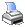Navigation:  System Specifications > Array SpecificationsAny field may be defined as being an array.  This means that each field is actually a multiple field.

Each array element is of the same type and size, and may be accessed using the same field name and just changing the element number.  The array element specifier is a numeric field, constant, or expression surrounded by squared brackets [] and immediately following (without any space separating) the field name.

TAS Professional allows only single dimensioned arrays; however, since the element number may be an expression you can create one that will treat the array as though it was multi-dimensioned.  The maximum number of elements in any one array is 4.3 billion (4gb); the first element in any array is 1.

EXAMPLE

SALES

SALES[MONTH[CNTR]]

COST_OF_GOODS[month(date())]

//The function month(date()) will return the month number from the current system date.

ARRAY[(cntr2-1)*24+cntr1]

(*The example above shows how a multi-dimensioned array might be handled.  The example assumes that cntr1 is the first dimension value and cntr2 is the second dimension counter and that there are 24 elements in each first dimension.  The limit of the second dimension is related to the total number of elements in the array only.*)